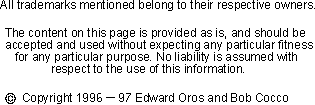Quarter Wave Line Matching Transformer

Some of the devices available to hams seem to have almost magical properties. The Q-section is one item that fits into that category.
What miracle does the Q-section perform? It has the ability to transform an input impedance into a different output impedance!
This means there are a variety of uses for the Q-section by hams, especially when it comes to antenna matching.
One use is in the matching of two transmission lines which have different impedances. For example, let's say you have a length of low loss 75 ohm cable that you want to use to feed your antenna. In order to match your 50 ohm cable to the 75 ohm cable, you'd need to insert a 1/4 wave section of transmission line between the two. Using the formula shown below, you'd find that the Q-section must have an impedance of 61.24 ohms.
Another use is in the matching of a driven element of a beam. Let's say you have a beam (split element type), with a feed point of 15 ohms. The square root of 15 (feed point) times 50 (normal coax cable) is 27.39 ohms. If you'd insert a 1/4 wave section of 27.39 ohm line, cut for the frequency of operation (between the antenna and your cable) you'd end up with a perfect match.
This page allows you to calculate the impedance required for a 1/4 wave (electrical) section of line.
To calculate the electrical length of the line you need to multiply the velocity factor of the line (available from the supplier of the line) by the free space length of 1/4 wave. For the length in feet, this is (245.9/frequency in MHz) * velocity. For the length in meters, this is (74.95/frequency in MHz) * velocity. See calculate electrical length of a line for more information
The formula for a quarter wave matching section is...
SQUARE ROOT( First Impedance To Match * Second Impedance To Match)
First Impedance
Second Impedance

Your new line must have an impedance of ohms

Back to the Antenna Elmer